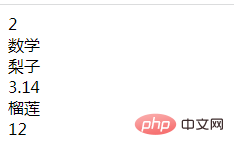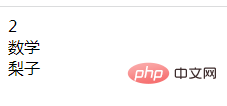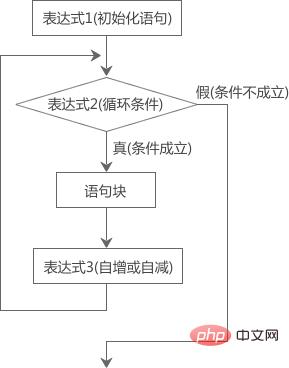# php怎么遍历数组只取前三个元素2023-03-18 06:48:01810浏览

php遍历数组只取前三个元素的方法：1、使用for语句循环遍历数组，语法“for(\$i=0; \$i<count(\$array); \$i++) {//循环体语句块;}”；2、在循环体中，使用if语句判断变量\$i是否大于2，大于就使用break语句跳出循环，不大于就继续输出元素，语法“if(\$i>2){break;}else{echo \$array[\$i]."<br/>";}”。• for语句用于遍历数组

• if语句用于限定遍历次数，获取前三个元素

for 循环遍历数组的语法格式如下：

```for (\$i=0; \$i< 数组长度; \$i++) {
//循环体语句块;
}```

```<?php
\$array= array(2,"数学","梨子",3.14,"榴莲",12);
for (\$i=0; \$i < count(\$array); \$i++) {
echo \$array[\$i] . "<br/>";
}
?>```• 如果大于，则表示遍历数组3次了，已获取了前三个元素，此时需要使用break语句跳出循环

• 如果不大于，则还没获取前三个元素，继续输出。

```<?php
\$array= array(2,"数学","梨子",3.14,"榴莲",12);
for (\$i=0; \$i < count(\$array); \$i++) {
if(\$i>2){
break;
}else{
echo \$array[\$i] . "<br/>";
}
}
?>```ok，可以看到输出了前三个元素。

for循环会将控制循环次数的变量预先定义在for语句中，因此for循环语句能够按照已知的循环次数进行循环操作，适用于明确知道脚本需要运行的次数的情况。

for 循环的语法格式如下：

```for (初始化语句; 循环条件; 变量更新--自增或自减) {
语句块;
}```

for循环语句可以拆解为4个部分：`()`号中的三个表达式和`{}`中的“语句块”，下面我们来分析一下。

• 初始化语句（表达式1）：主要是初始化一个变量值，用于设置一个计数器，即循环开始的值；该语句仅在第一次循环时执行，以后都不会再执行。

• 循环条件（表达式2）：循环执行的限制条件，用于控制是否执行循环体中的代码；如果条件为TRUE，则循环继续，如果条件为FALSE ，则循环结束，立即退出循环。

• 变量更新（表达式3）：一个带有自增或自减操作的表达式，循环每执行一次，马上修改计数器的值，以使循环条件逐渐变得“不成立”。

• 语句块：条件判断为真时，需要执行的若干代码。```<?php
\$sum=0;
for(\$i=1; \$i<=100; \$i++){
\$sum+=\$i;
}
echo '1 + 2 + 3 +...+ 99 + 100 = '. \$sum;
?>```

`1 + 2 + 3 +...+ 99 + 100 = 5050`

```<?php
\$sum=1;
for(\$i=1; \$i<=100; \$i++){
\$sum*=\$i;
}
echo '1 * 2 * 3 *...* 99 * 100 = '. \$sum;
?>```

`1 * 2 * 3 *...* 99 * 100 = 9.3326215443944E+157`